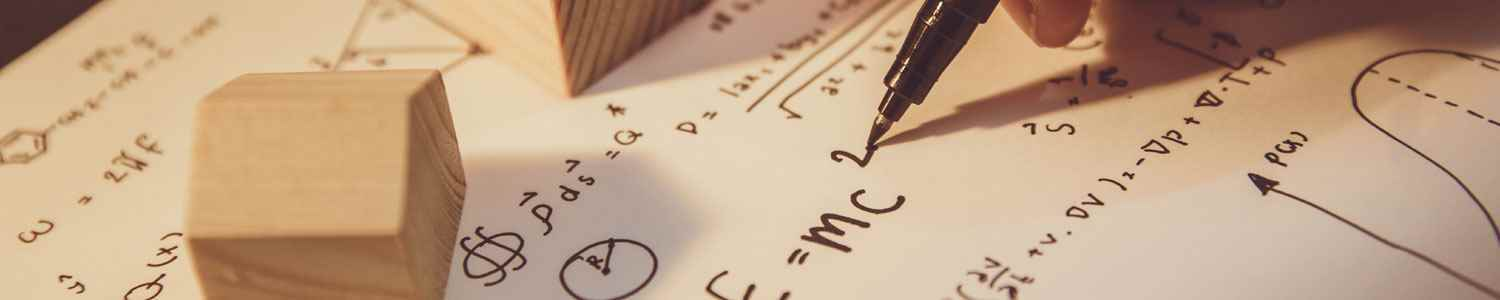# Mathematics and Statistics# Math Majors and Programs

Applied Mathematics

Provides students with the foundational and functional knowledge to apply math theory and techniques to solve complex problems. Involves computational methods, application of algorithms and numerical analysis, so computer knowledge can also be helpful in this area.

Mathematics

Pure math majors focus on algebra, geometry, calculus and discrete math.  These majors often end up in teaching, finance, computing and insurance.

Computer Science

While not directly a math major, mathematical principles are applied to a degree in Computer Science, whether it is developing algorithms, working with operating systems, data structures or theory.

Statistics

Stats majors learn how to work with data—how to college, analyze and interpret it—to inform practice and present data-based arguments.

Cryptography

This field involves making and breaking codes.  The obvious employers in this area are the government agencies like the NSA and CIA, but encoding is used in many areas such a data protection and telecommunications.

Biotechnology/Mathematical Biology

Mathematical biology has become a subject of interest due to recent breakthroughs in the study of DNA and proteins.

Operations Research

Operations Research uses analytical methods of problem solving and decision-making to assist in the management of organizations. INFORMS defines it as the scientific process of transforming data into insights to making better decisions.

Mathematical Physics

Developing mathematical methods to apply to problems within physics, or the mathematical foundations of theoretical physics.

## Mathematics and Statistics

### Applied Mathematics

Applied Mathematics, General
A program that focuses on the application of mathematics and statistics to the solution of functional problems in fields such as engineering and the applied sciences. Includes instruction in natural phenomena modeling continuum mechanics, reaction-diffusion, wave propagation, dynamic systems, numerical analysis, controlled theory, asymptotic methods, variation, optimization theory, inverse problems, and applications to specific scientific and industrial topics.

Computational and Applied Mathematics
A program that focuses on the application of a broad range of mathematical and computational methods to modeling, analysis, algorithm development, and simulation for the solution of complex scientific and engineering problems. Includes instruction in numerical analysis, discrete mathematics, operations research, optimization, differential equations, statistics, scientific computation, and applications to specific scientific and industrial topics.

Computational Mathematics
A program that focuses on the application of mathematics to the theory, architecture, and design of computers, computational techniques, and algorithms. Includes instruction in computer theory, cybernetics, numerical analysis, algorithm development, binary structures, combinatorics, advanced statistics, and related topics.

Financial Mathematics
A program that focuses on the application of mathematics and statistics to the finance industry, including the development, critique, and use of various financial models. Includes instruction in probability theory, statistical analysis, numerical methods, computation and simulation methods, stochastic processes, economics, and financial markets and applications.

Mathematical Biology
A program that focuses on the application of mathematics to genomics, molecular modeling, structural biology, ecology, evolutionary biology and systems of analysis of neurobiology, physiology, and metabolism. Includes instruction in computational mathematics, chaos and nonlinear systems, perturbation methods, evolutionary biology, molecular biology, bioinformatics, and neurobiology.

### Mathematics

Algebra and Number Theory
A program that focuses on the expression of quantities and their relationships by means of symbols, vectors, matrices, and equations, and the properties of integers. Includes instruction in algebraic structures, quadratic and automorphic forms, combinatorics, linear algebra, and algebraic geometry.

Analysis and Functional Analysis
A program that focuses on the properties and behavior of equations, multivariate solutions, functions, and dynamic systems. Includes instruction in differential equations, variation, approximations, complex variables, integrals, harmonic analysis and wavelet theory, dynamic systems, and applications to mathematical physics.

Geometry/Geometric Analysis
A program that focuses on the properties, measurements, and relationships pertaining to points, lines, angles, surfaces, and solids. Includes instruction in global analysis, differential geometry, Euclidian and Non-Euclidian geometry, set theory, manifolds, integral geometry, and applications to algebra and other topics.

Mathematics, General
A general program that focuses on the analysis of quantities, magnitudes, forms, and their relationships, using symbolic logic and language. Includes instruction in algebra, calculus, functional analysis, geometry, number theory, logic, topology and other mathematical specializations.

Topology and Foundations
A program that focuses on the properties of unaltered geometric configurations under conditions of continuous, multi-directional transformations. Includes instruction in mathematical logic, proof theory, model theory, set theory, combinatorics, continua, homotopy, homology, links, and transformation actions.

### Statistics

Mathematical Statistics and Probability
A program that focuses on the mathematical theory underlying statistical methods and their use. Includes instruction in probability theory parametric and non-parametric inference, sequential analysis, multivariate analysis, Bayesian analysis, experimental design, time series analysis, resampling, robust statistics, limit theory, infinite particle systems, stochastic processes, martingales, Markov processes, and Banach spaces.

Mathematics and Statistics
A program with a general synthesis of mathematics and statistics or a specialization which draws from mathematics and statistics. Includes instruction in calculus, linear algebra, numerical analysis and partial differential equations, discrete mathematics, probability theory, statistics, computing, and other related topics.

Statistics, General
A general program that focuses on the relationships between groups of measurements, and similarities and differences, using probability theory and techniques derived from it. Includes instruction in the principles in probability theory, binomial distribution, regression analysis, standard deviation, stochastic processes, Monte Carlo method, Bayesian statistics, non-parametric statistics, sampling theory, and statistical techniques.